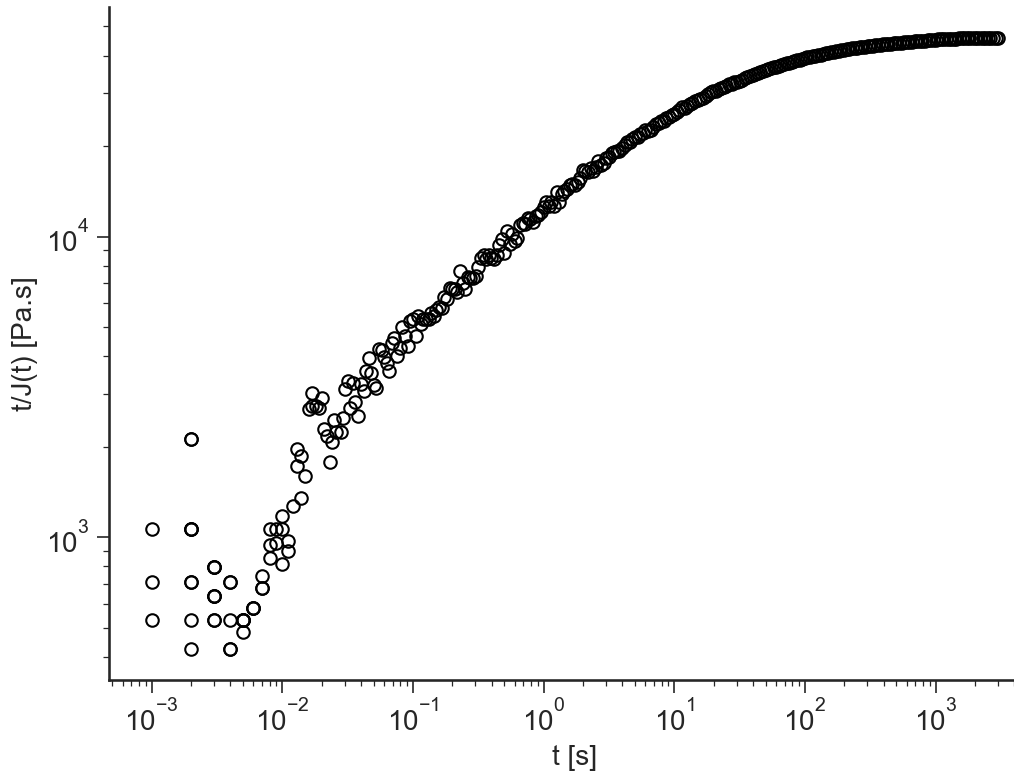# Creep: General description¶

## Purpose¶

Application to Analyze Data from Creep experiments

## Data Files¶

• The first line of the file should contain the sample parameters separated by semi-colons (;). It may contain any number of parameters which will be read and saved as file-parameter in RepTate.

• Then the data columns should appear, separated by spaces or tabs.

### .creep extension¶

Text files with .creep extension should be organised as follows:

• .creep files should contain at least the parameter value for the:

1. Applied stress stress

• 2 columns separated by spaces or tabs containing respectively:

1. time, $$t$$,

2. strain, $$\gamma$$,

A correct .creep file looks like:

stress=10;chem=PE;label=CM3;T=150;
t         strain          stress      T
s         -           Pa          C
1E-3  1.413E-5        10          149.9
1E-3  -9.419E-6       10          149.9
1E-3  -2.826E-5       10          149.9
...   ...         ...     ...


## Views¶

### log(gamma(t))¶

BaseApplicationCreep.viewLogStraint()[source]

Logarithm of the applied strain $$\gamma(t)$$ vs logarithm of time $$t$$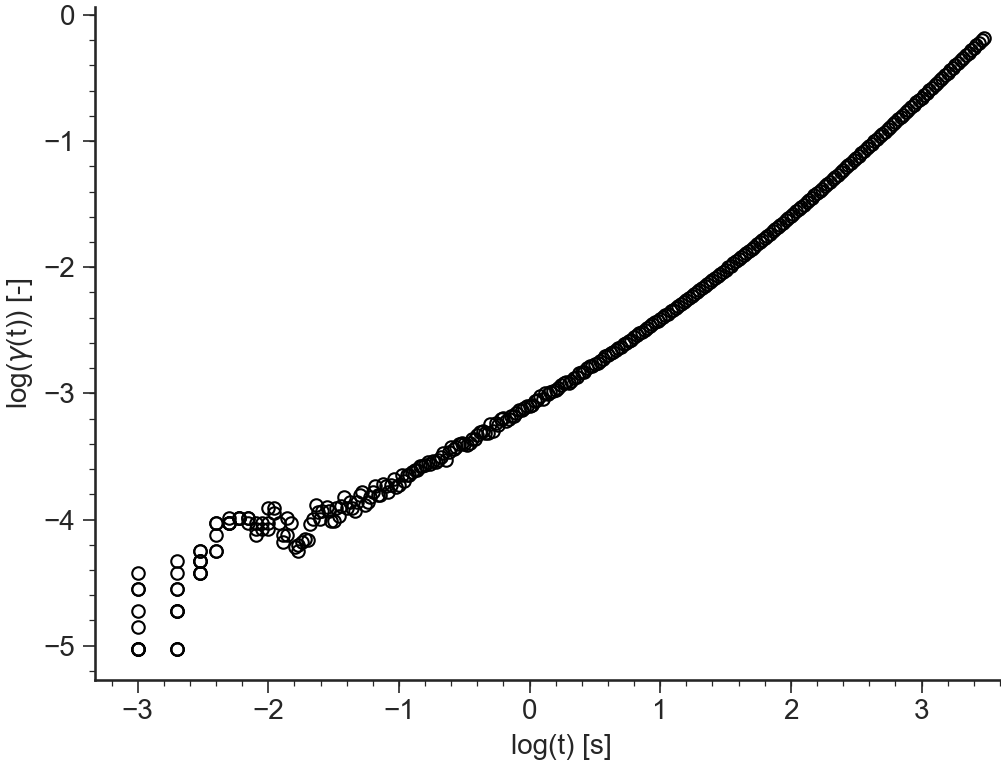### gamma(t)¶

BaseApplicationCreep.viewStraint()[source]

Applied strain $$\gamma(t)$$ vs time $$t$$ (both axes in logarithmic scale)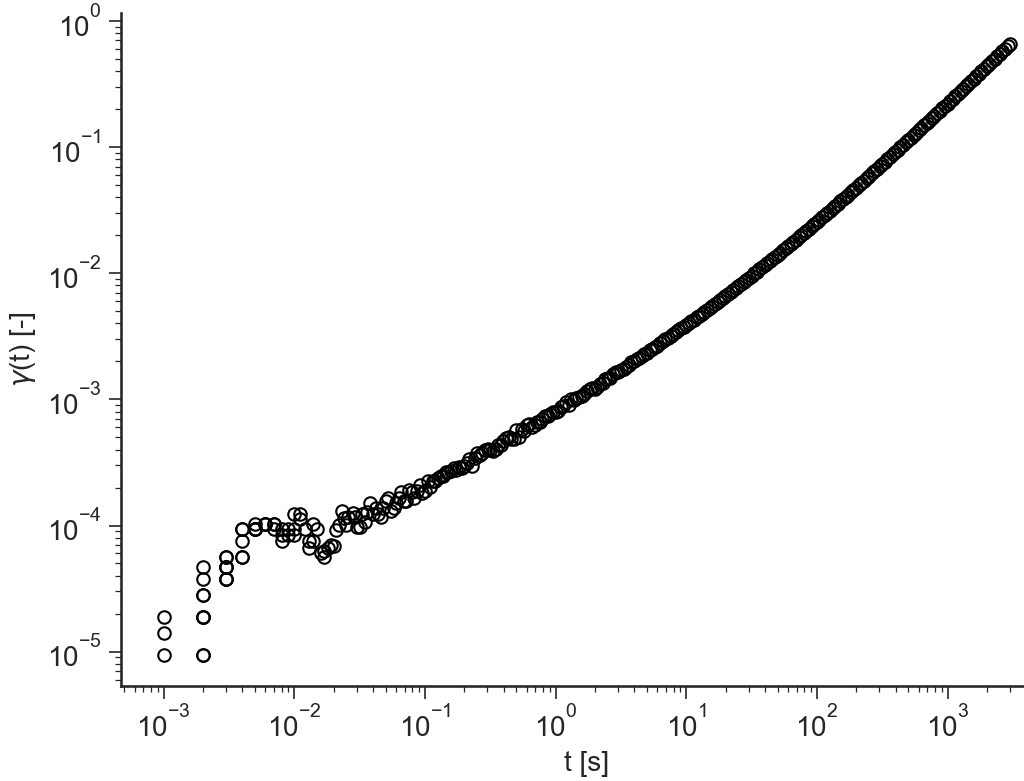### log(J(t))¶

BaseApplicationCreep.viewLogJt()[source]

Logarithm of the compliance $$J(t)=\gamma(t)/\sigma_0$$ (where $$\sigma_0$$ is the applied stress in the creep experiment) vs logarithm of time $$t$$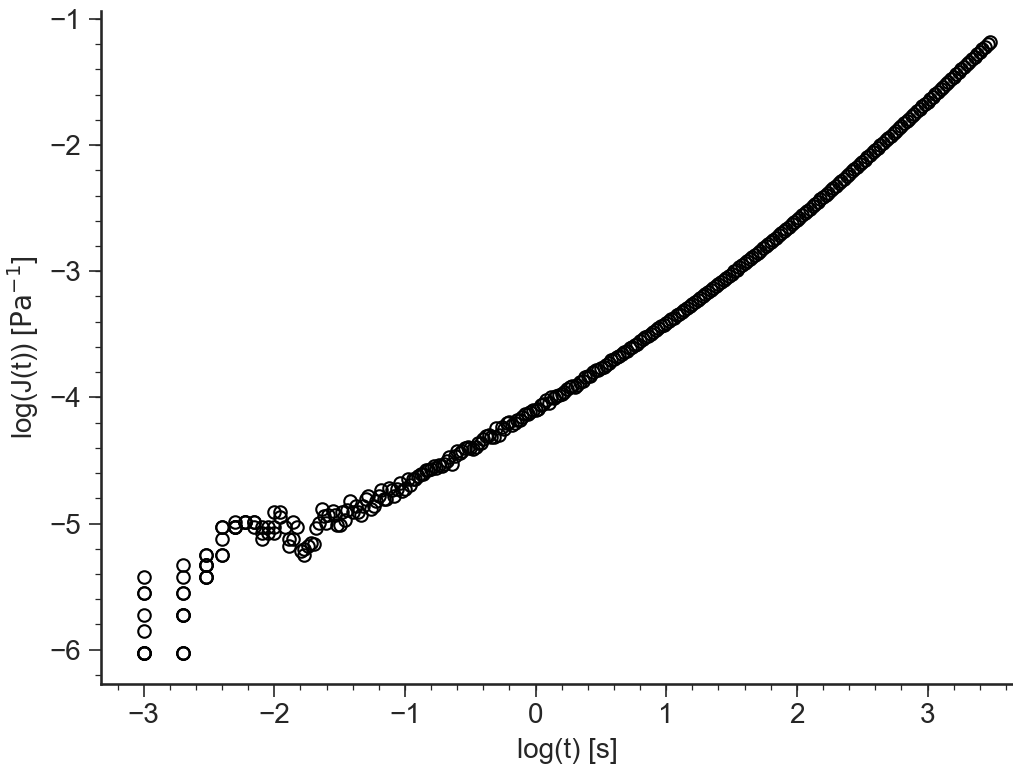### J(t)¶

BaseApplicationCreep.viewJt()[source]

Compliance $$J(t)=\gamma(t)/\sigma_0$$ (where $$\sigma_0$$ is the applied stress in the creep experiment) vs time $$t$$ (both axes in logarithmic scale)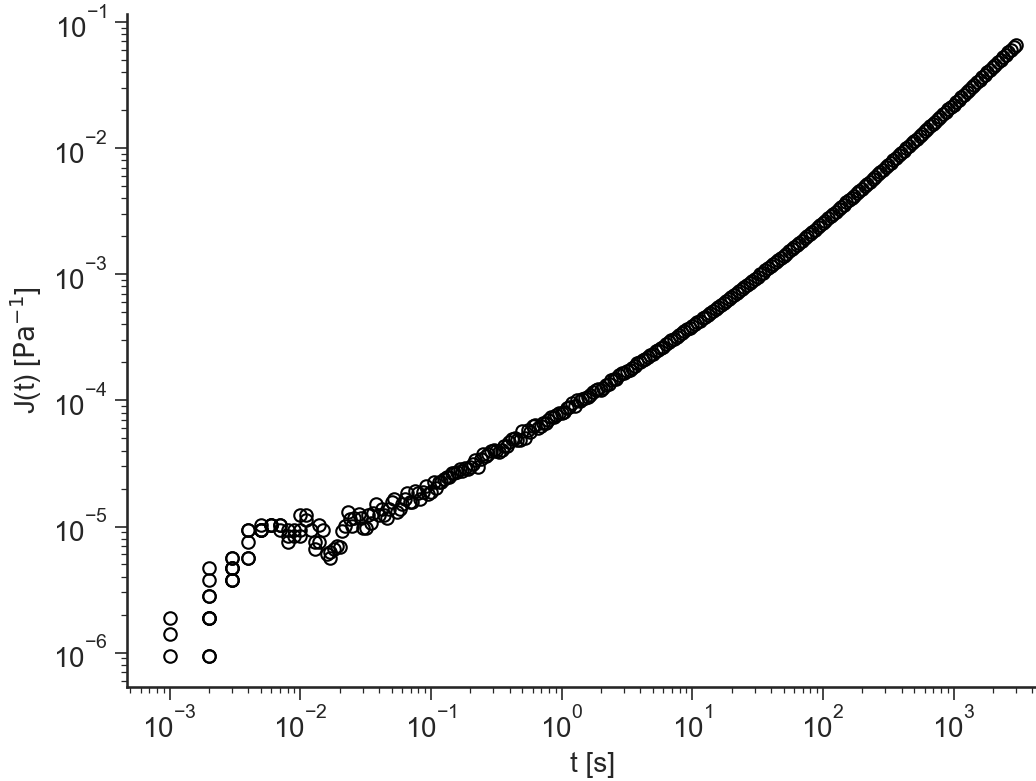### t/J(t)¶

BaseApplicationCreep.viewt_Jt()[source]

Time divided by compliance $$t/J(t)$$ vs time $$t$$ (both axes in logarithmic scale)